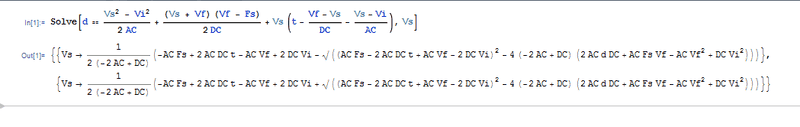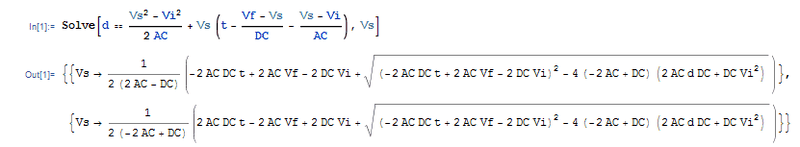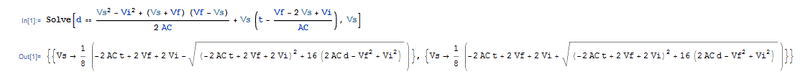# Calculating peak velocity

• larsbentley
In summary, the author is trying to program a motion control device to move a motor in a specific direction with a trapezoidal motion profile. They need to be able to calculate the peak velocity, and have an equation for calculating the distance traveled. They were advised to put the equation in quadratic form and isolate the Vs variable, but found it difficult.f

#### larsbentley

I am trying to program some motion control devices which have trapezoidal motion profiles to define a move. I can define the slewing(peak) velocity, accel/decel rates and distance to travel, the the hardware moves a motor the appropriate distance with the parameters given.

I need to be able to define a specific amount of time that a move will last, and calculate what the peak velocity should be when all other factors are known. Starting velocity is not always zero, but ending velocity will always zero for my purposes. The move will always be trapezoidal.

I have an equation for defining the distance traveled when all other factors are known, but I need to isolate the peak velocity to one side of the equation and I am not sharp enough at this math to be able to solve for the peak velocity. Any help would be greatly appreciated.

Assuming:
d = total distance traveled
t = total time
AC = acceleration
DC = deceleration
Vi = initial velocity
Vs = slewing (peak) velocity
Vf = final velocity

I have this equation which I believe is correct. I need to isolate Vs to one side:

d = [(Vs+Vi)/2]*[(Vs-Vi)/AC] + [(Vs+Vf)/2]*[(Vf-Fs)/DC] + Vs*[t-((Vf-Vs)/DC)-((Vs-Vi)/AC)]

Thanks

First here is a slightly cleaner way of presenting your equation
$$d = \frac{Vs^2 - Vi^2}{2AC} + \frac{(Vs + Vf)(Vf - Fs)}{2DC} + Vs ( t - \frac{Vf - Vs}{DC} - \frac{Vs - Vi}{AC} )$$

I then plugged this into Mathematica, the result seemed a bit hairy to type out here so I've attached it as an image.Dear JHamm,

Thank you so much for doing that for me... although I screwed up an would ask you or someone else to plug this equation back into Mathematica for me. I realized when trying to apply the equation you gave that I had included a typo in my original post. There is in fact no "Fs" variable, that was supposed to read "Vs". I have provided the corrected equation below. If you could re-run it for me as soon as possible I would greatly appreciate it.

Thanks much - Lars

corrected:

d = [(Vs+Vi)/2]*[(Vs-Vi)/AC] + [(Vs+Vf)/2]*[(Vf-Vs)/DC] + Vs*[t-((Vf-Vs)/DC)-((Vs-Vi)/AC)]

(sorry I tried to put it into the MathJax format but I couldn't seem to get it to work...)

Last edited:
Great! Thanks so much. I'll take a look at the guide.

Could I trouble you once more? I would like to see (Vs) solved for using just a portion of the equation, leaving off the deceleration period (the middle portion of the first equation):

$$d = \frac{Vs^2 - Vi^2}{2AC} + Vs ( t - \frac{Vf - Vs}{DC} - \frac{Vs - Vi}{AC} )$$

and then using only the acceleration portion:
$$d = \frac{Vs^2 - Vi^2}{2AC}$$

Thanks.

No problem :)
For the first one...And for the second...:)

Thanks! One more question :)

In the original equation you posted there is what I interpret as a Square Root symbol right about in the middle of the equation. I read this as if it extends to the end of the equation from there, based on the placement of parentheses.

In the new results you posted the square root symbols extend graphically over the portion of the equation that they are meant to cover. Is this just a quirk in the graphical output of Mathematica, or am I reading the first equation wrong?

Thanks

I would take it as a quirk, it seems as though if the expression becomes too long it will just use parenthesis instead :)

OK great, I will proceed accordingly. I have plugged the original equation into the motion control software I'm using, and I immediately see a problem. If AC = DC then I get a division by zero error, because the first item in the equation [ 1/2(ac-dc) ] will always equal zero if AC(acceleration) is equal to DC(deceleration), which is often the case.

I will need to set up my code to check and see if AC=DC, and if it does I will need to use an alternate equation. Would you mind running the original equation one more time for me, except replace all the DC variables with AC. I think this will get me what I need and keep the division by zero error from occurring.

Thanks again!

No problem mate :)Hope everything works out for you :)